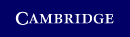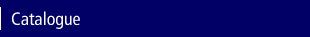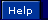Home > Catalogue > Origami, Eleusis, and the Soma Cube## Details

• 7 b/w illus.
• Page extent: 248 pages
• Size: 216 x 138 mm
• Weight: 0.39 kg

### Hardback

(ISBN-13: 9780521756105)

• Also available in Paperback
• ### For price and ordering options, inspection copy requests, and reading lists please select:

Please note local prices may applyView Excerpt as PDF (475KB)
Origami, Eleusis, and the Soma Cube
Cambridge University Press
9780521756105 - Origami, Eleusis, and the Soma Cube - Martin Gardner's Mathematical Diversions - By Martin Gardner
Excerpt

## Chapter One    The Five Platonic Solids

A REGULAR POLYGON is a plane figure bounded by straight lines, with equal sides and equal interior angles. There is of course an infinite number of such figures. In three dimensions the analog of the regular polygon is the regular polyhedron: a solid bounded by regular polygons, with congruent faces and congruent interior angles at its corners. One might suppose that these forms are also infinite, but in fact they are, as Lewis Carroll once expressed it, “provokingly few in number.” There are only five regular convex solids: the regular tetrahedron, hexahedron (cube), octahedron, dodecahedron, and icosahedron (see Figure 1).

The first systematic study of the five regular solids appears to have been made by the ancient Pythagoreans. They believed that the tetrahedron, cube, octahedron, and icosahedron respectively underlay the structure of the traditional four elements: fire, earth, air, and water. The dodecahedron was obscurely identified with the entire universe. Because these notions were elaborated in Plato's Timaeus, the regular polyhedrons came to be known as the Platonic solids. The beauty and fascinating mathematical properties of these five forms haunted scholars from the time of Plato through the Renaissance. The analysis of the Platonic solids provides the climactic final book of Euclid's Elements. Johannes Kepler believed throughout his life that the orbits of the six planets known in his day could be obtained by nesting the five solids in a certain order within the orbit of Saturn. Today the mathematician no longer views the Platonic solids with mystical reverence, but their rotations are studied in connection with group theory, and they continue to play a colorful role in recreational mathematics. Here we shall quickly examine a few diversions in which they are involved.

 Image not available in HTML version
Figure 1. The five Platonic solids. The cube and octahedron are “duals” in the sense that if the centers of all pairs of adjacent faces on one are connected by straight lines, the lines form the edges of the other. The dodecahedron and icosahedron are dually related in the same way. The tetrahedron is its own dual. (Artist: Bunji Tagawa)

 Image not available in HTML version
Figure 2. How a sealed envelope can be cut for folding into a tetrahedron. (Artist: Bunji Tagawa)

There are four different ways in which a sealed envelope can be cut and folded into a tetrahedron. The following is perhaps the simplest. Draw an equilateral triangle on both sides of one end of an envelope (see Figure 2). Then cut through both layers of the envelope as indicated by the broken line and discard the right-hand piece. By creasing the paper along the sides of the front and back triangles, points A and B are brought together to form the tetrahedron.

Figure 3 shows the pattern for a tantalizing little puzzle currently marketed in plastic. You can make the puzzle yourself by cutting two such patterns out of heavy paper. (All the line segments except the longer one have the same length.) Fold each pattern along the lines and tape the edges to make the solid shown. Now try to fit the two solids together to make a tetrahedron. A mathematician I know likes to annoy his friends with a practical joke based on this puzzle. He bought two sets of the plastic pieces so that he could keep a third piece concealed in his hand. He displays a tetrahedron on the table, then knocks it over with his hand and at the same time releases the concealed piece. Naturally his friends do not succeed in forming the tetrahedron out of the three pieces.

Concerning the cube, I shall mention only an electrical puzzle and the surprising fact that a cube can be passed through a hole in a smaller cube. If you will hold a cube so that one corner points

 Image not available in HTML version
Figure 3. A pattern (left) that can be folded into a solid (right), two of which make a tetrahedron. (Artist: Bunji Tagawa)
directly toward you, the edges outlining a hexagon, you will see at once that there is ample space for a square hole that can be slightly larger than the face of the cube itself. The electrical puzzle involves the network depicted in Figure 4. If each edge of the cube has a resistance of one ohm, what is the resistance of the entire structure when current flows from A to B? Electrical engineers have been known to produce pages of computations on this problem, though it yields easily to the proper insight.

All five Platonic solids have been used as dice. Next to the cube the octahedron seems to have been the most popular. The pattern shown in Figure 5, its faces numbered as indicated, will fold into a

 Image not available in HTML version
Figure 4. An electrical-network puzzle. (Artist: Bunji Tagawa)

 Image not available in HTML version
Figure 5. A strip to make an octahedral die. (Artist: Bunji Tagawa)
neat octahedron whose open edges can be closed with transparent tape. The opposite sides of this die, as in the familiar cubical dice, total seven. Moreover, a pleasant little mind-reading stunt is made possible by this arrangement of digits. Ask someone to think of a number from 0 to 7 inclusive. Hold up the octahedron so that he sees only the faces 1, 3, 5, and 7, and ask him if he sees his chosen number. If he says “Yes,” this answer has a key value of 1. Turn the solid so that he sees faces 2, 3, 6, and 7, and ask the question again. This time “Yes” has the value of 2. The final question is asked with the solid turned so that he sees 4, 5, 6, and 7. Here a “Yes” answer has the value of 4. If you now total the values of his three answers you obtain the chosen number, a fact that should be easily explained by anyone familiar with the binary system. To facilitate finding the three positions in which you must hold the solid, simply mark in some way the three corners that must be pointed toward you as you face the spectator.

There are other interesting ways of numbering the faces of an octahedral die. It is possible, for example, to arrange the digits 1 through 8 in such a manner that the total of the four faces around each corner is a constant. The constant must be 18, but there are three distinct ways (not counting rotations and reflections) in which the faces can be numbered in this fashion.

An elegant way to construct a dodecahedron is explained in Hugo Steinhaus's book Mathematical Snapshots. Cut from heavy cardboard two patterns like the one pictured at left in Figure 6. The pentagons should be about an inch on a side. Score the outline of each center pentagon with the point of a knife so that the pentagon flaps fold easily in one direction. Place the patterns together as shown at right in the illustration so that the flaps of each pattern fold toward the others. Weave a rubber band alternately over

 Image not available in HTML version
Figure 6. Two identical patterns are fastened together with a rubber band to make a pop-up dodecahedron. (Artist: Bunji Tagawa)
and under the projecting ends, keeping the patterns pressed flat. When you release the pressure, the dodecahedron will spring magically into shape.

If the faces of this model are colored, a single color to each face, what is the minimum number of colors needed to make sure that no edge has the same color on both sides? The answer is four, and it is not difficult to discover the four different ways that the colors can be arranged (two are mirror images of the other two). The tetrahedron also requires four colors, there being two arrangements, one a reflection of the other. The cube needs three colors and the octahedron two, each having only one possible arrangement. The icosahedron calls for three colors; here there are no less than 144 different patterns, only six of which are identical with their mirror images.

If a fly were to walk along the 12 edges of an icosahedron, traversing each edge at least once, what is the shortest distance it could travel? The fly need not return to its starting point, and it would be necessary for it to go over some edges twice. (Only the octahedron's edges can be traversed without retracing.) A plane projection of the icosahedron (Figure 7) may be used in working on this problem, but one must remember that each edge is one unit in length. (I have been unable to resist concealing a laconic Christmas greeting in the

 Image not available in HTML version
Figure 7. A plane projection of an icosahedron. (Artist: Bunji Tagawa)
way the corners of this diagram are labeled. It is not necessary to solve the problem in order to find it.)

In view of the fact that cranks persist in trying to trisect the angle and square the circle long after these feats have been proved impossible, why has there been no comparable effort to find more than five regular polyhedrons? One reason is that it is quite easy to “see” that no more are possible. The following simple proof goes back to Euclid.

A corner of a polyhedron must have at least three faces. Consider the simplest face: an equilateral triangle. We can form a corner by putting together three, four, or five such triangles. Beyond five, the angles total 360 degrees or more and therefore cannot form a corner. We thus have three possible ways to construct a regular convex solid with triangular faces. Three and only three squares will similarly form a corner, indicating the possibility of a regular solid with square faces. The same reasoning yields one possibility with three pentagons at each corner. We cannot go beyond the pentagon, because when we put three hexagons together at a corner, they equal 360 degrees.

This argument does not prove that five regular solids can be constructed, but it does show clearly that no more than five are

possible. More sophisticated arguments establish that there are six regular polytopes, as they are called, in four-dimensional space. Curiously, in every space of more than four dimensions there are only three regular polytopes: analogs of the tetrahedron, cube, and octahedron.

A moral may be lurking here. There is a very real sense in which mathematics limits the kinds of structures that can exist in nature. It is not possible, for example, that beings in another galaxy gamble with dice that are regular convex polyhedra of a shape unknown to us. Some theologians have been so bold as to contend that not even God himself could construct a sixth Platonic solid in three-dimensional space. In similar fashion, geometry imposes unbreakable limits on the varieties of crystal growth. Some day physicists may even discover mathematical limitations to the number of fundamental particles and basic laws. No one of course has any notion of how mathematics may, if indeed it does, restrict the nature of structures that can be called “alive.” It is conceivable, for example, that the properties of carbon compounds are absolutely essential for life. In any case, as humanity braces itself for the shock of finding life on other planets, the Platonic solids serve as ancient reminders that there may be fewer things on Mars and Venus than are dreamt of in our philosophy.

### Answers

The total resistance of the cubical network is 5/6 ohm. If the three corners closest to A are short-circuited together, and the same is done with the three corners closest to B, no current will flow in the two triangles of short circuits because each connects equipotential points. It is now easy to see that there are three one-ohm resistors in parallel between A and the nearest triangle (resistance 1/3 ohm), six in parallel between the triangles (1/6 ohm), and three in parallel between the second triangle and B (1/3 ohm), making a total resistance of 5/6 ohm.

C. W. Trigg, discussing the cubical-network problem in the November–December 1960 issue of Mathematics Magazine, points out that a solution for it may be found in Magnetism and Electricity, by E. E. Brooks and A. W. Poyser, 1920. The problem and the method

of solving it can be easily extended to networks in the form of the other four Platonic solids.

The three ways to number the faces of an octahedron so that the total around each corner is 18 are 6, 7, 2, 3 clockwise (or counterclockwise) around one corner, and 1, 4, 5, 8 around the opposite corner (6 adjacent to 1, 7 to 4, and so on); 1, 7, 2, 8 and 4, 6, 3, 5; and 4, 7, 2, 5 and 6, 1, 8, 3. See W. W. Rouse Ball's Mathematical Recreations and Essays, Chapter 7, for a simple proof that the octahedron is the only one of the five solids whose faces can be numbered so that there is a constant sum at each corner.

The shortest distance the fly can walk to cover all edges of an icosahedron is 35 units. By erasing five edges of the solid (for example, edges FM, BE, JA, ID, and HC) we are left with a network that has only two points, G and K, where an odd number of edges come together. The fly can therefore traverse this network by starting at G and going to K without retracing an edge – a distance of 25 units. This is the longest distance it can go without retracing. Each erased edge can now be added to this path, whenever the fly reaches it, simply by traversing it back and forth. The five erased edges, each gone over twice, add 10 units to the path, making a total of 35.

### Postscript

Margaret Wertheim, writing on “A Puzzle Finally Makes the ‘Cosmic Figures’ Fit,” in The New York Times (May 10, 2005), describes a remarkable puzzle created by Dr. Wayne Daniel, a retired physicist living in Genoa, Nevada. Called All Five, it consists of 41 wooden pieces that form the five Platonic solids, all nested together like Russian matryoshka dolls. Outside is the icosahedron, followed by the dodecahedron, cube, tetrahedron, and in the center a tiny octahedron. There are no empty spaces between the pieces! Dr. Daniel has constructed other puzzles based on the five regular solids, but this one is his crowning achievement. He has made a DVD showing how the pieces come apart and go back together. It can be seen on his Web site.

In the books to follow in this series, there are many references to problems and curiosities involving the five solids. Note in particular

a chapter in Book 10 on Jean Pedersen's way of plaiting polyhedra with paper strips, and references there cited. A chapter devoted entirely to tetrahedra is in Book 5.

One can imagine how amazed and delighted Plato and Kepler would have been if someone had given them an All Five.

© Cambridge University Press